# Exercise 1: Computation of equivalent units under weighted average and FIFO method

## Exercise 1 (a):

The Mexico Company manufactures a single product that goes through two departments. The data relating to activity performed in first department during the month of June is given below: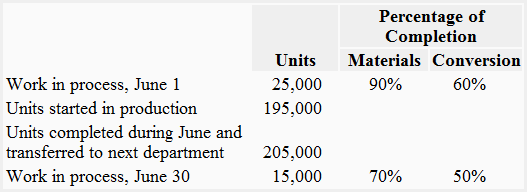Required: Compute the equivalent units of production assuming the company uses:

1. weighted average method
2. FIFO method

### Solution

#### 1. Equivalent units of production – weighted average method: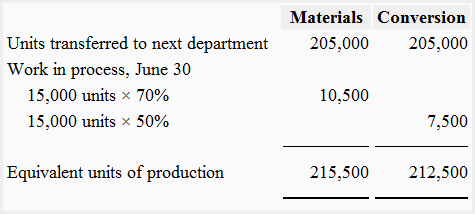#### 2. Equivalent units of production – FIFO method: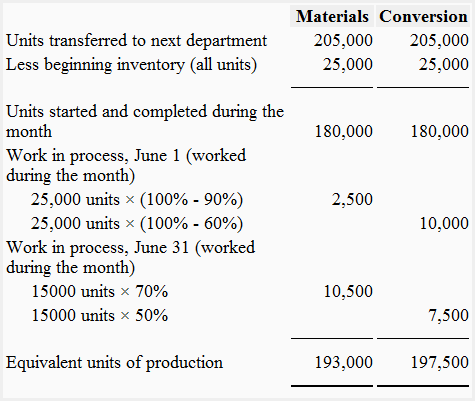A D V E R T I S E M E N T

## Exercise 1 (b):

The following data has been collected from three different situations. Units transferred out are 100% complete with respect to all cost elements. Stages of completion of beginning and ending inventories are applicable to all cost elements.

1. Started in process: 26,000 units
Transferred out: 22,000 units
Work in process ending inventory: 1,600 units 1/2 completed and 2,400 units 1/4 completed
2. Work in process beginning inventory: 25,000 units, 2/5 completed
Started in process: 100,000 units
Transferred out: 105,000 units
Work in process ending inventory: 12,000 units 1/2 completed and 8,000 units 1/4 completed
3. Work in process beginning inventory: 18,000 units 1/3 completed and 12,000 units 1/2 completed
Started in process: 75,000 units
Transferred out: 90,000 units
Work in process ending inventory: 6,000 units 1/2 completed and 9,000 units 1/3 completed

Required: Compute equivalent production in each of the above situations using:

1. weighted average costing method
2. FIFO costing method

### Solution

#### 1. Equivalent production under weighted average method: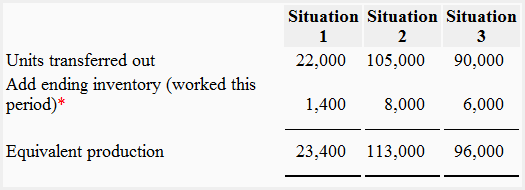*Equivalent units in ending inventory:

The equivalent units in work in process ending inventory consist of the percentage of work done in current period. The computations of equivalent units in work in process ending inventory for all three situations are given below:

Situation 1:
= (1600 units × 1/2) + (2400 units × 1/4)
= 800 units + 600 units
= 1,400 units

Situation 2:
= (12,000 units × 1/2) + (8,000 units × 1/4)
= 6,000 units + 2,000 units
= 8,000 units

Situation 3:
= (6,000 units × 1/2) + (9000 units × 1/3)
= 3,000 units + 3,000 units
= 6,000 units

#### 2. Equivalent production under FIFO method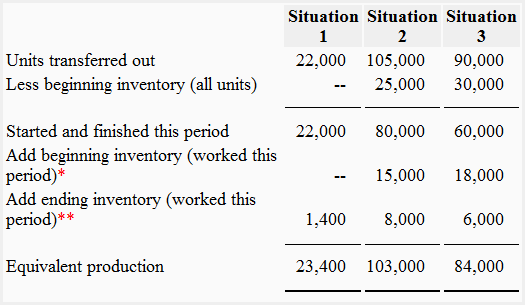*Equivalent units in beginning inventory:

The equivalent units in work in process beginning inventory consist of the percentage of work done in current period. The computations of equivalent units in work in process beginning inventory for all three situations are given below:

Situation 1:
There is no beginning inventory in situation 1.

Situation 2:
= 25,000 units × 3/5
= 15,000 units

Situation 3:
= (18,000 units × 2/3) + (12,000 units × 1/2)
= 12,000 units + 6,000 units
= 18,000 units

**Equivalent units in ending inventory:

The equivalent units in work in process ending inventory consist of the percentage of work done in current period. The computations of equivalent units in work in process ending inventory for FIFO method are the same as under weighted average method.

Situation 1:
= (1600 units × 1/2) + (2400 units × 1/4)
= 800 units + 600 units
= 1,400 units

Situation 2:
= (12,000 units × 1/2) + (8,000 units × 1/4)
= 6,000 units + 2,000 units
= 8,000 units

Situation 3:
= (6,000 units × 1/2) + (9000 units × 1/3)
= 3,000 units + 3,000 units
= 6,000 units

Show your love for us by sharing our contents.

### One Comment on Exercise 1: Computation of equivalent units under weighted average and FIFO method

1.Shreshth

Great exercise and good explanation